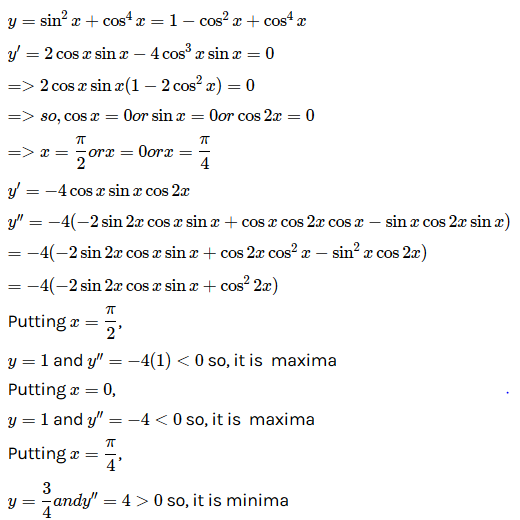×#### Thank you for registering.

One of our academic counsellors will contact you within 1 working day.

Click to Chat

1800-1023-196

+91-120-4616500

CART 0

• 0

MY CART (5)

Use Coupon: CART20 and get 20% off on all online Study Material

ITEM
DETAILS
MRP
DISCOUNT
FINAL PRICE
Total Price: Rs.

There are no items in this cart.
Continue Shopping
```
Given A=sin^2x+cos^4x,then for all real x, min≤A≤max
Given A=sin^2x+cos^4x,then for all real x, min≤A≤max

```
3 years ago

```							We Know thatSin^2x Also Cos^2x >= Cos^4xAdd sin^2x both sidesCos^2x + sin^2x >= Cos^4x + sin^2x 1 >= Cos^4x + sin^2xTherefore max of A is 1Also both terms  Cos^4x + sin^2x= (1 – sin^2x)^2 + sin^2x= 1 + sin^4x – 2sin^2x + sin^2x=Sin^4x – sinn^2x + 1 = (sin^2x – 1/2)^2  + 3/4now Min =3/4 and Max = 1
```
3 years ago
```							Dear Student,Please find the solution to your problem in the attached image.Thanks and Regards```
6 months ago
Think You Can Provide A Better Answer ?

## Other Related Questions on Trigonometry

View all Questions »### Course Features

• 731 Video Lectures
• Revision Notes
• Previous Year Papers
• Mind Map
• Study Planner
• NCERT Solutions
• Discussion Forum
• Test paper with Video Solution### Course Features

• 31 Video Lectures
• Revision Notes
• Test paper with Video Solution
• Mind Map
• Study Planner
• NCERT Solutions
• Discussion Forum
• Previous Year Exam Questions